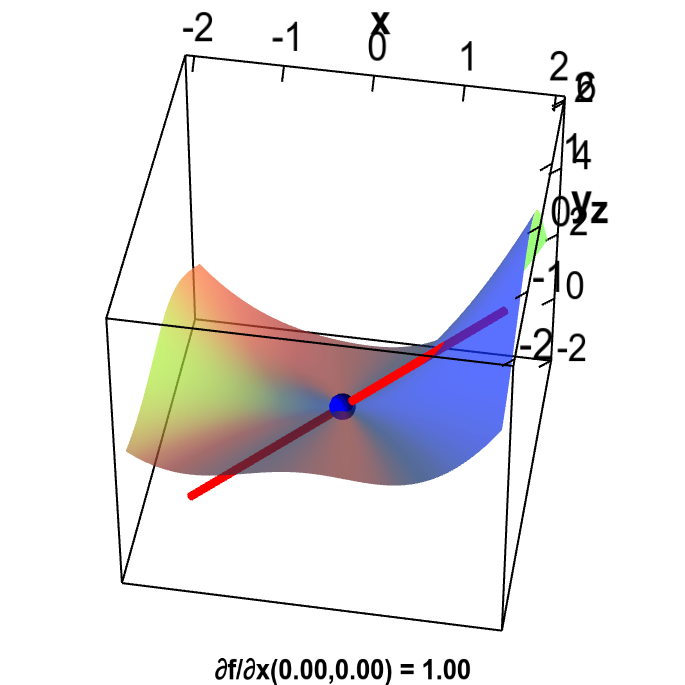# Math Insight

### Applet: Example partial derivative by limit definintionThe partial derivative of a function $f(x,y)$ at the origin is illustrated by the red line that is tangent to the graph of $f$ in the $x$ direction. The partial derivative $\pdiff{f}{x}(0,0)$ is the slope of the red line. The partial derivative at $(0,0)$ must be computed using the limit definition because $f$ is defined in a piecewise fashion around the origin: \begin{align*} f(x,y) = \begin{cases} \displaystyle \frac{x^3 +x^4-y^3}{x^2+y^2} & \text{if } (x,y) \ne (0,0)\\ 0 & \text{if } (x,y) = (0,0). \end{cases} \end{align*}

You can change the point $(x,y)$ at which $\pdiff{f}{x}(x,y)$ is evaluated by dragging the blue point. Since $\pdiff{f}{x}(x,y)$ is discontinuous around $(0,0)$, the derivative jumps as soon as you move the point.